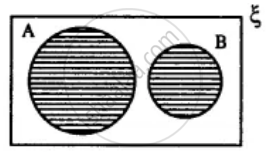# Two Sets a and B Are Such that a ∩ B = φ. Draw a Venn-diagram to Show the Relationship Between a and B. Shade the Region Representing : a ∪ B - Mathematics

Diagram

Two sets A and B are such that A ∩ B = Φ. Draw a venn-diagram to show the relationship between A and B. Shade the region representing :
A ∪ B

#### Solution

A ∪ B =Concept: Venn Diagram
Is there an error in this question or solution?

#### APPEARS IN

Selina Concise Mathematics Class 8 ICSE
Chapter 6 Sets
Exercise 6 (E) | Q 7.1 | Page 76